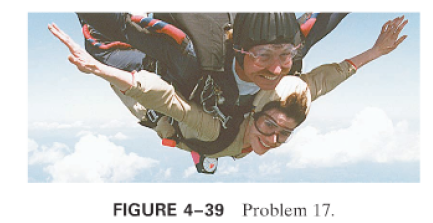×
Get Full Access to Physics: Principles With Applications - 6 Edition - Chapter 4 - Problem 17p
Get Full Access to Physics: Principles With Applications - 6 Edition - Chapter 4 - Problem 17p

×

# (II) (a) What is the acceleration of two falling skyISBN: 9780130606204 3

## Solution for problem 17P Chapter 4

Physics: Principles with Applications | 6th Edition

• Textbook Solutions
• 2901 Step-by-step solutions solved by professors and subject experts
• Get 24/7 help from StudySoup virtual teaching assistantsPhysics: Principles with Applications | 6th Edition

4 5 1 324 Reviews
24
5
Problem 17P

(II) (a) What is the acceleration of two falling sky divers (massincluding parachute) when the upward force of air resistance is equal to one-fourth of their weight? () After popping open the parachute, the divers descend leisurely to the ground at constant speed. What now is the force of air resistance on the sky divers and their parachute? See Fig..Step-by-Step Solution:

Solution 17P: The force (friction) due to air is responsible of lowering the net force due to gravity and with the help of parachute the skydivers can be brought safely on the ground. Step 1 of 3 Concept: Newton’s second law: The force F acts on mass m produces an acceleration a in the object. Mathematically, F=ma.

Step 2 of 4

Step 3 of 4

##### ISBN: 9780130606204

This full solution covers the following key subjects: parachute, divers, air, resistance, sky. This expansive textbook survival guide covers 35 chapters, and 3914 solutions. The full step-by-step solution to problem: 17P from chapter: 4 was answered by , our top Physics solution expert on 03/03/17, 03:53PM. Since the solution to 17P from 4 chapter was answered, more than 2021 students have viewed the full step-by-step answer. This textbook survival guide was created for the textbook: Physics: Principles with Applications, edition: 6. Physics: Principles with Applications was written by and is associated to the ISBN: 9780130606204. The answer to “?(II) (a) What is the acceleration of two falling sky divers (mass including parachute) when the upward force of air resistance is equal to one-fourth of their weight? ( ) After popping open the parachute, the divers descend leisurely to the ground at constant speed. What now is the force of air resistance on the sky divers and their parachute? See Fig. .” is broken down into a number of easy to follow steps, and 63 words.

Unlock Textbook Solution